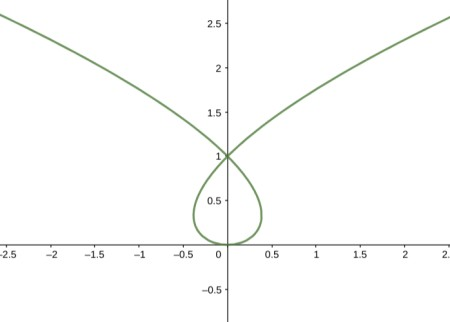# Let C be the curve defined by the parametric equation x=t^{3} - t, and y =t^{2}. (a)Show that C...

## Question:

Let {eq}C{/eq} be the curve defined by the parametric equation {eq}x=t^{3} - t{/eq}, and {eq}y =t^{2}{/eq}.

(a)Show that {eq}C{/eq} has two tangents at the point {eq}(0, 1){/eq} and find their equations.

(b)Find the points on {eq}C{/eq} where the tangent is horizontal or vertical.

(c)Determine where the curve is concave upward or downward.

(d)Sketch {eq}C{/eq}.

## Parametric Curves

A parametric curve {eq}\displaystyle x=x(t), y=y(t), a\leq t\leq b {/eq} has a direction, which is given by the trace on the curve when the value of t is increasing.

The slope of the tangent line to the parametric curve given above is calculated as

{eq}\displaystyle \frac{dy}{dx}(t)=\frac{y'(t)}{x'(t)}, {/eq} where {eq}\displaystyle '=\frac{d}{dt}. {/eq}

The curve is concave up when the second derivative is positive and concave down, when the second derivative is negative.

The second derivative is calculated as before,

{eq}\displaystyle \frac{d^2y}{dx^2}=\frac{d}{dt}\left(\frac{y'(t)}{x'(t)}\right)\cdot \frac{1}{x'(t)}. {/eq}

(a) To show that the curve {eq}\displaystyle x=t^3-t, y=t^2, {/eq} has two tangents at {eq}\displaystyle (0,1), {/eq}

we will obtain the slope of the tangent lines to the given curve.

Before, we need to find the parameter t corresponding to the given point,

{eq}\displaystyle x=0=t^3-t=t(t^2-1)\text{ and } y=1 =t^2 \implies t^2=1\iff t=-1 \text{ or } t=1. {/eq}

The slope of the tangent line requires the following derivatives:

{eq}\displaystyle x'(t) =\frac{d}{dt}(t^3-t)=3t^2-1 \displaystyle y'(t) =\frac{d}{dt}(t^2)=2t {/eq}

So, the two slopes of the tangents lines correspond to the two parameters, t,

{eq}\displaystyle \boxed{\frac{y'(t)}{x'(t)}\bigg\vert_{t=-1}=-1 \text{ and } \frac{y'(t)}{x'(t)}\bigg\vert_{t=1}=1}. {/eq}

The two tangent lines are given as {eq}\displaystyle y=y(-1)+\frac{y'}{x'}(-1)(x-x(-1))\iff \boxed{y=1-x}\\ \displaystyle y=y(1)+\frac{y'}{x'}(1)(x-x(1))\iff \boxed{y=1+x}. {/eq}

(b) To find the points that admit horizontal tangent lines, we will solve

{eq}\displaystyle y'(t)=0, \text{ such that } x'(t)\neq 0 \implies 2t=0 \implies t=0 \text{ and } x'(0)=(3t^2-1)\bigg\vert_{t=0}=-1\neq 0 \\ \displaystyle \implies \boxed{ \text{ the point that admits horizontal tangent lines is } t=0 \text{ or } (0,0)}. {/eq}

To find the points that admit vertical tangent lines, we will solve

{eq}\displaystyle x'(t)=0, \text{ such that } y'(t)\neq 0 \implies 3t^2-1=0 \implies t=\pm \frac{1}{\sqrt{3}} \text{ and } y'\left(\pm \frac{1}{\sqrt{3}}\right)\neq 0\\ \displaystyle \implies \boxed{ \text{ the points that admit vertical tangent lines are } t=- \frac{1}{\sqrt{3}} \text{ or } \left(-3^{3/2}+3^{-1/2}, 3^{-1}\right), t=\frac{1}{\sqrt{3}} \text{ or } \left(3^{3/2}-3^{-1/2}, 3^{-1}\right)}. {/eq}

c) To determine the values of {eq}\displaystyle t, {/eq} where the parametric curve concave up or down, we need to calculate the second derivative.

{eq}\displaystyle \begin{align} \frac{d^2y}{dx^2}&=\frac{d}{dt}\left(\frac{y'(t)}{x'(t)}\right)\cdot \frac{1}{x'(t)}\\ &=\frac{d}{dt}\left(\frac{2t}{3t^2-1}\right)\cdot \frac{1}{3t^2-1} \\ &=-2\frac{ 3t^2+1}{(3t^2-1)^3} \end{align} {/eq}

Therefore, the curve is concave up when {eq}\displaystyle \frac{d^2y}{dx^2}>0 \iff 3t^2-1<0 \text{ ( because } 3t^2+1>0 \text{ for all values} ) \iff \boxed{t\in\left(- \frac{1}{\sqrt{3}}, \frac{1}{\sqrt{3}}\right)}. {/eq}

and the curve is concave down when {eq}\displaystyle \frac{d^2y}{dx^2}<0 \iff 3t^2-1>0 \iff \boxed{t\in\left(-\infty, - \frac{1}{\sqrt{3}}\right)\cup\left( \frac{1}{\sqrt{3}}, \infty\right)}. {/eq}

(d) The curve is plotted below with the green solid line, showing the point (0,1) that admits two different tangent lines.